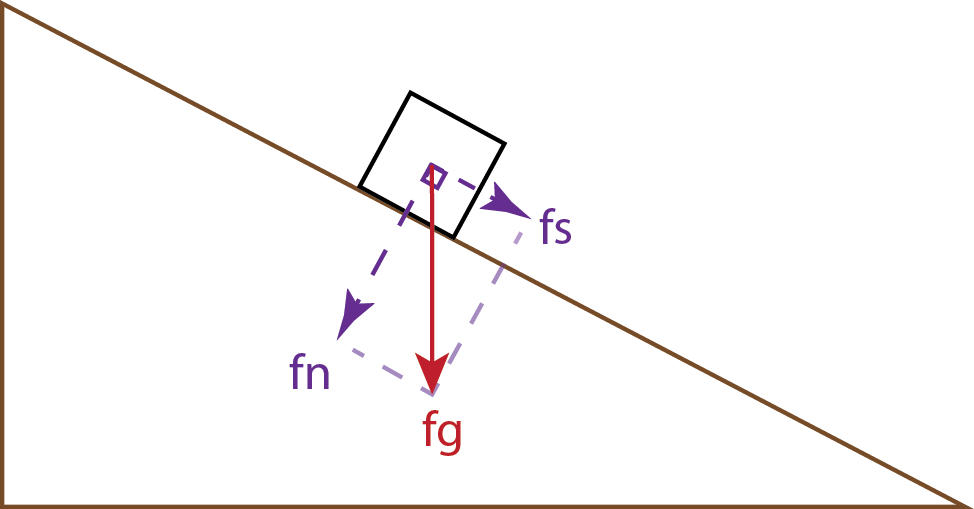# 8.1: Slope Strength

•• Chris Johnson, Matthew D. Affolter, Paul Inkenbrandt, & Cam Mosher
• Salt Lake Community College via OpenGeology
$$\newcommand{\vecs}{\overset { \rightharpoonup} {\mathbf{#1}} }$$ $$\newcommand{\vecd}{\overset{-\!-\!\rightharpoonup}{\vphantom{a}\smash {#1}}}$$$$\newcommand{\id}{\mathrm{id}}$$ $$\newcommand{\Span}{\mathrm{span}}$$ $$\newcommand{\kernel}{\mathrm{null}\,}$$ $$\newcommand{\range}{\mathrm{range}\,}$$ $$\newcommand{\RealPart}{\mathrm{Re}}$$ $$\newcommand{\ImaginaryPart}{\mathrm{Im}}$$ $$\newcommand{\Argument}{\mathrm{Arg}}$$ $$\newcommand{\norm}{\| #1 \|}$$ $$\newcommand{\inner}{\langle #1, #2 \rangle}$$ $$\newcommand{\Span}{\mathrm{span}}$$ $$\newcommand{\id}{\mathrm{id}}$$ $$\newcommand{\Span}{\mathrm{span}}$$ $$\newcommand{\kernel}{\mathrm{null}\,}$$ $$\newcommand{\range}{\mathrm{range}\,}$$ $$\newcommand{\RealPart}{\mathrm{Re}}$$ $$\newcommand{\ImaginaryPart}{\mathrm{Im}}$$ $$\newcommand{\Argument}{\mathrm{Arg}}$$ $$\newcommand{\norm}{\| #1 \|}$$ $$\newcommand{\inner}{\langle #1, #2 \rangle}$$ $$\newcommand{\Span}{\mathrm{span}}$$$$\newcommand{\AA}{\unicode[.8,0]{x212B}}$$Figure $$\PageIndex{1}$$: Forces on a block on an inclined plane (fg = force of gravity; fn = normal force; fs = shear force).

Mass wasting occurs when a slope fails. A slope fails when it is too steep and unstable for existing materials and conditions. Slope stability is ultimately determined by two principal factors: the slope angle and the strength of the underlying material. Force of gravity, which plays a part in mass wasting, is constant on the Earth’s surface for the most part, although small variations exist depending on the elevation and density of the underlying rock. In the figure, a block of rock situated on a slope is pulled down toward the Earth’s center by the force of gravity (fg). The gravitational force acting on a slope can be divided into two components: the shear or driving force (fs) pushing the block down the slope, and the normal or resisting force (fn) pushing into the slope, which produces friction. The relationship between shear force and normal force is called shear strength. When the normal force, i.e., friction, is greater than the shear force, then the block does not move downslope. However, if the slope angle becomes steeper or if the earth material is weakened, shear force exceeds normal force, compromising shear strength, and downslope movement occurs.Figure $$\PageIndex{1}$$: As slope increases, the force of gravity (fg) stays the same and the normal force decreases while the shear force proportionately increases.

In the figure, the force vectors change as the slope angle increases. The gravitational force doesn’t change, but the shear force increases while the normal force decreases. The steepest angle at which rock and soil material is stable and will not move downslope is called the angle of repose. The angle of repose is measured relative from the horizontal. When a slope is at the angle of repose, the shear force is in equilibrium with the normal force. If the slope becomes just slightly steeper, the shear force exceeds the normal force, and the material starts to move downhill. The angle of repose varies for all material and slopes depending on many factors such as grain size, grain composition, and water content. The figure shows the angle of repose for sand that is poured into a pile on a flat surface. The sand grains cascade down the sides of the pile until coming to rest at the angle of repose. At that angle, the base and height of the pile continue to increase, but the angle of the sides remains the same.Figure $$\PageIndex{1}$$: Angle of repose in a pile of sand.

Water is a common factor that can significantly change the shear strength of a particular slope. Water is located in pore spaces, which are empty air spaces in sediments or rocks between the grains. For example, assume a dry sand pile has an angle of repose of 30 degrees. If water is added to the sand, the angle of repose will increase, possibly to 60 degrees or even 90 degrees, such as a sandcastle being built at a beach. But if too much water is added to the pore spaces of the sandcastle, the water decreases the shear strength, lowers the angle of repose, and the sandcastle collapses.

Another factor influencing shear strength are planes of weakness in sedimentary rocks. Bedding planes (see Chapter 5) can act as significant planes of weakness when they are parallel to the slope but less so if they are perpendicular to the slope . locations A and B, the bedding is nearly perpendicular to the slope and relatively stable. At location D, the bedding is nearly parallel to the slope and quite unstable. At location C, the bedding is nearly horizontal, and the stability is intermediate between the other two extremes . Additionally, if clay minerals form along bedding planes, they can absorb water and become slick. When a bedding plane of shale (clay and silt) becomes saturated, it can lower the shear strength of the rock mass and cause a landslide, such as at the 1925 Gros Ventre, Wyoming rock slide. See the case studies section for details on this and other landslides.Figure $$\PageIndex{1}$$: Locations A and B have bedding nearly perpendicular to the slope, making for a relatively stable slope. Location D has bedding nearly parallel to the slope, increasing the risk of slope failure. Location C has bedding nearly horizontal and the stability is relatively intermediate.

This page titled 8.1: Slope Strength is shared under a CC BY-NC-SA 4.0 license and was authored, remixed, and/or curated by Chris Johnson, Matthew D. Affolter, Paul Inkenbrandt, & Cam Mosher (OpenGeology) via source content that was edited to the style and standards of the LibreTexts platform; a detailed edit history is available upon request.